Sample Scripts Filters as Lua scripts for Project Dogwaffle & PD ProSample Lua Scripts.......

Ok, here are some filters, for your enjoyment. Grab'em and experiment, play with different parameters, etc...

 Filter Description Submitted by Flag of Gambia New! a step by step tutorial Philip Staiger Flag of Switzerland (and France) New! a simple script, great for beginning coders Philip Staiger Water Reflection adapted from a script seen on the ArtWeaver lua forum Philip Staiger Polar texture mapping - based on Paul Bourke's algorithm & info Philip Staiger Grid - render a grid, with colors (with GUI) Dan Ritchie Mirror - Mirrors both horizontally and verttically Dan Ritchie www.squirreldome.com Slider - slides some rows (depending on a random choice), refreshes the display and then repeats the process Marco Pontello www.mark0.net Mirrored frame - puts a small frame within the border of the image, which is based on mirrored components of the image.  More scripts at artweaver.de. Boris Eyrich www.artweaver.de Sharpen Luminance - This lua script applies a sharpen convolution to the luminance channel of an image. Dan Ritchie Elevation - "raise" a pixel diagonally depending on its value Marco Pontello HAM - Hold and Modify, from the the good ole' Amiga days Dan Ritchie and Marco Pontello Value-only Contrast Dan Ritchie NV - Night Vision Dan Ritchie Soften_Chroma Dan Ritchie DesatGrad_YUV Dan Ritchie Channels_HSV_to_RGB Dan Ritchie Channels_rgb_to_hsv Dan Ritchie replace_a_color - look for all pixels of a given color and replace them with a new color Philip Staiger replace_a_color_range  - replace pixels with a new color: now you can also specify a range for r/g/b values Philip Staiger & Dan Ritchie Jitter Blur Dan Ritchie DuoTone Dan Ritchie Gamma Filter Dan Ritchie Wave Deformation Filter Dan Ritchie Fractal Noise Dan Ritchie Shift RGB Dan Ritchie Halftone (with GUI) Dan Ritchie InvertValue Dan Ritchie Dynamic Range Dan Ritchie Channels_RGB_to_CMY_cyan Dan Ritchie Channels_RGB_to_CMY_yellow Dan Ritchie Channels_RGB_to_CMY_magenta Dan Ritchie

Mirror - by Dan Ritchie

my first lua script. ok, it's not terribly complex.

Copy/Paste the text shown here into the DogLua editing window >>>
or save it to a .lua file to run it from the DogLuaBrowser.

left: original     /     right: Mirror'ed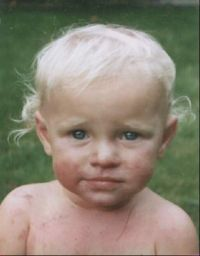>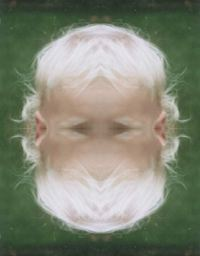-- Mirror_by_Dan.lua -- Mirror.  Mirrors both horiz and vert... for y = 0, height - 1 do   for x = 0, width - 1 do     x2=(width/2)-math.abs(x-(width/2))     y2=(height/2)-math.abs(y-(height/2))     r, g, b = get_rgb(x2, y2)     set_rgb(x, y, r, g, b)   end   progress(y / height) end

Sliders - by Marco Pontello

Here's a script that slides some rows (depending on a random choice), refreshes the display and then repeats the process a selected number of times. The resulting effect maybe similar to something reflected on water, or something like that.

Anyway, it is just to show how to put in a function for some repeating blocks/process, ask for user input, commit the buffers modification and update the display while continuing the process.

Copy/Paste the text shown here into the DogLua editing window >>>
or save it to a .lua file to run it from the DogLuaBrowser.

left: Slider'ed     /     right: Mirrored, Shifted, Alpha-Slider'ed
(i.e. with use of Alpha channel mask to work on bottom areas)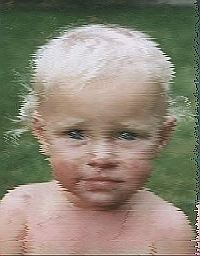>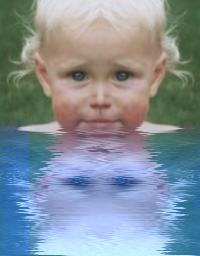-- Sliders_by_Marco.lua function slide(y)   back_r, back_g, back_g = get_rgb(0,y)   for x = 0, bound_x1 - 1 , 1 do     r, g, b = get_rgb(x + 1, y)     set_rgb(x, y, r, g, b)   end   set_rgb(width - 1, y, back_r, back_g, back_g) end rep = Dog_ValueBox("Slider", "Repeat:", 1, 50, 5) prob = Dog_ValueBox("Slider", "Probability:", 1, 20, 5) counter = 0 maxcounter = rep * (bound_y1 - bound_y0) for t = 1, rep, 1 do   for y = bound_y0, bound_y1, 1 do     c = math.random(prob)     if c == 1 then       slide(y)     end     counter = counter + 1     progress(counter / maxcounter)   end   flush()   Dog_Refresh() end --

Mirrored Frame - by Boris Eyrichshown with permission by Boris Eyrich, Artweaver.de:-- mirrored_frame.lua FrameSize = 30 function InsideFrame(x,y)   if (x > FrameSize) and (x < width-FrameSize-1) and      (y > FrameSize) and (y < height-FrameSize-1) then     return 1   else     return 0   end end for y=0, height-1 do   for x=0, width-1 do     if InsideFrame(x,y) == 0 then       r,g,b,a = get_rgba(width-x-1,height-y-1)     else       r,g,b,a = get_rgba(x,y)     end     set_rgba(x,y,r,g,b,a)   end   progress (y/height) end

Sharpen Luminance, by Dan Ritchie

This lua script applies a sharpen convolution to the luminance channel of an image.  Save the script as Sharpen_Luminance.lua

This script will give you a somewhat better sharpen than the regular sharpen convolution.  The digital photo enhance filter will still generally give you better results, but use this if you need more extreme sharpening.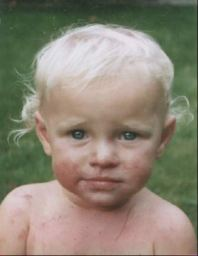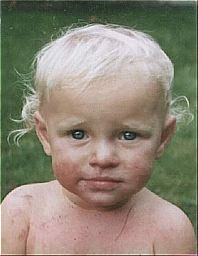Sharpen_Luminance, before (l.) and after (r.)
 -- Sharpen_Luminance.lua -- apply a sharpening convolution to the value channel. kernel = { { -1, -1,  -1},            { -1,  12,  -1},            { -1, -1,  -1} } divisor = 4 offset = 0 function filter (x,y)    local i, j, sum, v2 , dummy1, dummy2    sum = 0    for i=-1,1 do       for j=-1,1 do          dummy1,dummy2,v2=get_hsv(x+i, y+j)          sum = sum + (kernel[j+2][i+2] * v2)       end    end    return (sum / divisor) + offset end for y=bound_y0, bound_y1-1 do    for x=bound_x0, bound_x1-1 do        h,s,v = get_hsv(x,y)        v = filter (x,y)        set_hsv (x,y,h,s,v)    end    progress ((y-bound_y0)/(bound_y1-bound_y0)) end

Elevation, by Marco Pontello

 -- Elevation -- "raise" a pixel diagonally depending on its value function elevate(x, y, h)   local i   r, g, b = get_rgb(x, y)   v = get_value(x, y)   for i = v * h, 1, -1 do     set_rgb(x+i, y-i, r, g, b)   end end factor = Dog_ValueBox("Elevation", "Factor:", 1, 100, 30) if factor > 0 then   for y = bound_y0, bound_y1 do     for x = bound_x1, bound_x0, -1 do       elevate(x, y, factor)     end     progress((y - bound_y0) / (bound_y1 - bound_y0))   end end

HAM (Hold and Modify) - remember the Amiga days?
by Dan Ritchie and Marco Pontello

The Ham script simulates the Amigas HAM (Hold and Modify) screen mode.

Install the .lua script and the bmp file in your DogLuaScripts folder.  Place the exe files in the folder where Dogwaffle is installed, if you do not already have them.

It is assumed that DogLua and the GUI_Server application are already installed.

This scripts comes with a choice of 4 dithering modes:

Floyd
Halftone
Random
None

Here is an example of the effect, with no dithering:

original image:  Plasma noise (level 7) - click image for larger version

modified image: HAM (dithering = None) - click image for larger version

Usage in 3D: Sandicastle 'terraced' terrain elevation map

The quantization produces nifty maps that can be used like elevation maps for 3D terrain generation such as the one found in Carrara Studio 3: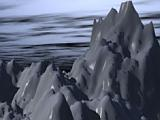before and after HAM effect: making sand castle mountains
click images for larger views

 -- HAM -- Simulate the H.A.M. (Hold And Modify) -- mode of the Amiga's Days! -- some functions... -- Determine the greater value of 3 function max_p(x1, x2, x3) local v if x1 > x2 then  if x1 > x3 then   v = 1  else   v = 3  end  else   if x2 > x3 then   v = 2  else   v = 3  end end return v end -- Quantize each component in 4bit range function quantize(x)  x = math.floor(x/16) * 16  return x end -- begin main program... GUI_SetCaption("Ham") GUI_AddLogo("HamLogo.bmp") --setup a combo box h1 = GUI_AddControl("Combobox", "Dithering") --set the list in the combo box GUI_SetList (h1,0,"Floyed") GUI_SetList (h1,1,"Halftone") GUI_SetList (h1,2,"Random") GUI_SetList (h1,3,"None") --set the current string in the combo box GUI_SetSettings (h1,0, "Floyed") GUI_OpenPanel() repeat idx, retval, retstr = GUI_WaitOnEvent() until idx < 0 -- if OK was pressed. if (idx==-1) then h1_value, h1_string = GUI_GetSettings (h1) --dither the image using and existing plugin if h1_string =="Floyed" then Dog_ShellExe("12_bit_dither_pf.exe") Dog_GetBuffer() end if h1_string =="Halftone" then Dog_ShellExe("12_bit_halftone_pf.exe") Dog_GetBuffer() end if h1_string =="Random" then Dog_ShellExe("12_bit_random_pf.exe") Dog_GetBuffer() end for y = bound_y0, bound_y1 do -- Start with black at every scanline lr = 0 lg = 0 lb = 0 for x = bound_x0, bound_x1 do r, g, b = get_rgb(x, y) r=r*255 g=g*255 b=b*255 r = quantize(r) g = quantize(g) b = quantize(b) r=r/255 g=g/255 b=b/255 -- Calculate the difference with last pixel dr = math.abs(r - lr) dg = math.abs(g - lg) db = math.abs(b - lb) -- Change only the most different component v = max_p(dr, dg, db) if v == 1 then lr = r elseif v == 2 then lg = g else lb = b end set_rgb(x, y, lr, lg, lb) end progress((y - bound_y0) / (bound_y1-bound_y0)) end end GUI_ClosePanel()

Value-only Contrast (by Dan Ritchie)

Here's another super simple script that's actually useful.  It
increases the contrast of an image without changing the colors.

 -- ValueOnly_contrast.lua -- value only contrast -keep colors the same. contrast = Dog_ValueBox("Contrast",         "0-49 less.  51-100 more.", 0, 100, 50) -- 0-1 will reduce contrast -- 1-2 will increase contrast contrast=(contrast/50) for y = 0, height - 1 do   for x = 0, width - 1 do     y2, u, v = get_yuv(x, y)     y2=((y2-.5)*contrast)+.5     set_yuv(x, y, y2, u, v)   end   progress(y / height) end

NV - Night Vision like effect

 --NV.lua -- Night vision like effect. -- uses the saturation channel, -- so it won't work with greyscale images. for y = 0, height - 1 do   for x = 0, width - 1 do     r, g, b = get_hsv(x, y) b=1-b b=b+(math.random()-.5)/5 if b<0 then b=0 end if b>1 then b=1 end     set_rgb(x, y, 0, b, 0)   end   progress(y / height) end

Soften_Chroma

 -- Soften_Chroma.lua -- apply a box filter to the U anv V channels. -- based on the 3x3 convolution filter example kernel = { { 1, 1, 1},            { 1, 1, 1},            { 1, 1, 1} } divisor = 9 offset = 0               function filter (x,y)    local i, j, sum, sum2    sum = 0    sum2= 0     local y2, u,v       for i=-1,1 do       for j=-1,1 do            y2,u,v=get_yuv(x+i, y+j)               sum = sum + (kernel[j+2][i+2] * u)          sum2 = sum2 + (kernel[j+2][i+2] * v)       end    end      return (sum / divisor) + offset, (sum2 / divisor) + offset end for y=bound_y0, bound_y1-1 do    for x=bound_x0, bound_x1-1 do        y2,u,v= get_yuv(x,y)             u,v = filter (x,y)        set_yuv (x,y,y2,u,v)    end    progress ((y-bound_y0)/(bound_y1-bound_y0)) end

 -- DesatGrad_YUV (looks better than HSV version) -- Desaturate gradient for y = 0, height - 1 do   for x = 0, width -1 do     h, s, v = get_yuv(x, y)     s = s * (x / width)     set_yuv(x, y, h, s, v)   end   progress(y / height) end

Channels_HSV_to_RGB

 -- Channels_HSV_to_RGB.lua for y = 0, height - 1 do   for x = 0, width - 1 do     r, g, b = get_rgb(x, y)     set_hsv(x, y, r, g, b)   end   progress(y / height) end

Channels_rgb_to_hsv

 --Channels_rgb_to_hsv.lua for y = 0, height - 1 do   for x = 0, width - 1 do     r, g, b = get_hsv(x, y)     set_rgb(x, y, r, g, b)   end   progress(y / height) end

replace_a_color

(see below for another version with range (margin) and user interface)

This version doesn't use any GUI components.  You can specify each color channel  as a normalized floating point value (0.0 to 1.0) . So if you're used to values from 0 (integer) to 255 simply take your value and divide it by 255.0

Of course the script could be changed instead, to accept user data ranging from 0 to 255. See the next script for an example.

 -- -- color_search_and_replace -- -- replace all pixels of a given 'bad' color -- with a partyicular new color. The bad color is -- defined by an r/g/b triplet. -- ...looking for this 'bad' color. Note that colors in -- gluas are ultimately normalized in the range between -- 0.0 and 1.0. If however you like to think of -- a color as a byte-value between 0 and 255 then -- you could divide that value by 255 to normalize it to -- a range of 0.0 to 1.0 -- for example: LOOKING FOR BLACK (zero in r/g/b each) bad_r = 0.0 bad_g = 0.0 bad_b = 0.0 -- ...and to replace it with this 'new' color: -- for example change to red (1.0 in r channel only) new_r = 1.0 new_g = 0.0 new_b = 0.0 -- now go through all pixels, check their value, and if it's -- a match then replace it with the new color for y = 0, height - 1 do   for x = 0, width - 1 do -- get the rgb values of the current pixel     r, g, b = get_rgb(x, y) -- check if it's the same as the 'bad' color we're looking for -- it's most likely not the case so we're looking one -- by one in order to avoid needless tests     if r == bad_r  then       if g == bad_g  then          if b == bad_b  then            set_rgb(x, y, new_r, new_g, new_b)          end       end         end   end   progress(y / height) end

replace_a_color_range

Similar to the prior script, but this one also lets you specify a margin. If a pixel is found to be of the same color or within the margin then it gets replaced with the new color.

In this example, the colors are specified as  8-bit integers ranging from 0 to 255. The script then normalizes the values to what's needed.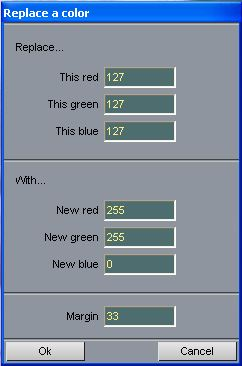Below are a few examples :
(with various settings, not necessarily the user options seen here to the right):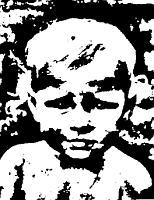original image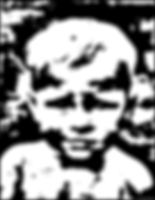... further modified with blurring...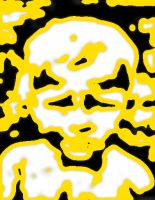... and finally replacing the blurred grey values with yellow. The prior blurring causes transitions between the original black and white. The range can be set to replace most of the greys with this one yellow color.

Jitter Blur (with GUI)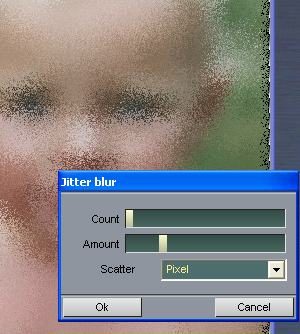-- Jitterblur_Gui.lua function DoJitter(count,amount) edge_duplicate=1 local x,y --math.randomseed(1) for y = 0, height-1 do     for x = 0, width-1 do        local hsum,ssum,vsum=0,0,0 local nx,ny,h,s,v,dummy        for n=1, count do           nx=x+(math.random()-0.5)*amount           ny=y+(math.random()-0.5)*amount           h,s,v= get_rgb(nx,ny)           hsum,ssum,vsum=hsum+h,ssum+s,vsum+v        end        set_rgb (x,y, hsum/count,ssum/count,vsum/count)     end     progress(y/height) end progress(0) end function DoJitterRGB(count,amount) edge_duplicate=1 local x,y --math.randomseed(1) for y = 0, height-1 do     for x = 0, width-1 do        local hsum,ssum,vsum=0,0,0 local nx,ny,h,s,v,dummy        for n=1, count do           nx=x+(math.random()-0.5)*amount           ny=y+(math.random()-0.5)*amount           h,dummy,dummy= get_rgb(nx,ny)           nx=x+(math.random()-0.5)*amount           ny=y+(math.random()-0.5)*amount           dummy,s,dummy= get_rgb(nx,ny)           nx=x+(math.random()-0.5)*amount           ny=y+(math.random()-0.5)*amount           dummy,dummy,v= get_rgb(nx,ny)           hsum,ssum,vsum=hsum+h,ssum+s,vsum+v        end        set_rgb (x,y, hsum/count,ssum/count,vsum/count)     end     progress(y/height) end progress(0) end function DoJitterHSV(count,amount) edge_duplicate=1 local x,y --math.randomseed(1) for y = 0, height-1 do     for x = 0, width-1 do        local hsum,ssum,vsum=0,0,0 local nx,ny,h,s,v,dummy        for n=1, count do           nx=x+(math.random()-0.5)*amount           ny=y+(math.random()-0.5)*amount           h,dummy,dummy= get_hsv(nx,ny)           nx=x+(math.random()-0.5)*amount           ny=y+(math.random()-0.5)*amount           dummy,s,dummy= get_hsv(nx,ny)           nx=x+(math.random()-0.5)*amount           ny=y+(math.random()-0.5)*amount           dummy,dummy,v= get_hsv(nx,ny)           hsum,ssum,vsum=hsum+h,ssum+s,vsum+v        end        set_hsv (x,y, hsum/count,ssum/count,vsum/count)     end     progress(y/height) end progress(0) end function DoJitterYUV(count,amount) edge_duplicate=1 local x,y --math.randomseed(1) for y = 0, height-1 do     for x = 0, width-1 do        local hsum,ssum,vsum=0,0,0 local nx,ny,h,s,v,dummy        for n=1, count do           nx=x+(math.random()-0.5)*amount           ny=y+(math.random()-0.5)*amount           h,dummy,dummy= get_yuv(nx,ny)           nx=x+(math.random()-0.5)*amount           ny=y+(math.random()-0.5)*amount           dummy,s,dummy= get_yuv(nx,ny)           nx=x+(math.random()-0.5)*amount           ny=y+(math.random()-0.5)*amount           dummy,dummy,v= get_yuv(nx,ny)           hsum,ssum,vsum=hsum+h,ssum+s,vsum+v        end        set_yuv (x,y, hsum/count,ssum/count,vsum/count)     end     progress(y/height) end progress(0) end -- the main program. Dog_SaveUndo() GUI_SetCaption("Jitter blur") h1 = GUI_AddControl("Scroller", "Count", 1, 1, 10) h2 = GUI_AddControl("Scroller", "Amount", 10, 1, 100) h3 = GUI_AddControl("Combobox", "Scatter") GUI_SetList (h3,0,"Pixel") GUI_SetList (h3,1,"RGB separately") GUI_SetList (h3,2,"HSV separately") GUI_SetList (h3,3,"YUV separately") GUI_SetSettings (h3,0, "Pixel") GUI_OpenPanel() --event loop.  Repeat until ok or cancel pressed (index of -1 or -2) repeat idx, retval, retstr = GUI_WaitOnEvent() count,string=GUI_GetSettings(h1) amount,string=GUI_GetSettings(h2) dummy,type=GUI_GetSettings(h3) if (idx>-1) then if type=="Pixel" then DoJitter (count,amount) end if type=="RGB separately" then DoJitterRGB (count,amount) end if type=="HSV separately" then DoJitterHSV (count,amount) end if type=="YUV separately" then DoJitterYUV (count,amount) end Dog_Refresh() end until idx < 0 --repeat until GUI_ClosePanel() if idx == -2 then --Restore the image Dog_RestoreUndo() Dog_GetBuffer() end

Channels_RGB_to_CMY_cyan
 -- Channels_RGB_to_CMY_cyan.lua -- Convert a RGB image to cyan local x, y, r, g, b local c2,m2,y2,av for y = 0, height - 1 do   for x = 0, width - 1 do     r, g, b = get_rgb(x, y) c2=r m2=1 y2=1     set_rgb(x, y, c2, m2, y2)   end   progress(y / height) end
Channels_RGB_to_CMY_magenta
 -- Channels_RGB_to_CMY_magenta.lua -- Convert a RGB image to magenta? local x, y, r, g, b local c2,m2,y2,av for y = 0, height - 1 do   for x = 0, width - 1 do     r, g, b = get_rgb(x, y) c2=1 m2=g y2=1     set_rgb(x, y, c2, m2, y2)   end   progress(y / height) end
Channels_RGB_to_CMY_yellow
 -- Channels_RGB_to_CMY_yellow.lua -- Convert a RGB image to Yellow? local x, y, r, g, b local c2,m2,y2,av for y = 0, height - 1 do   for x = 0, width - 1 do     r, g, b = get_rgb(x, y) c2=1 m2=1 y2=b     set_rgb(x, y, c2, m2, y2)   end   progress(y / height) end
Dynamic Range
 -- Dynamic Range.lua -- expand the dynamic range to the full 8 bit range. local x, y, r, g, b,min,max,mu --find the min and max values in the image min=256 max=-1 for y = 2, height - 3 do   for x = 2, width - 3 do     r, g, b = get_rgb(x, y)     if rmax then max=r end     if gmax then max=g end     if bmax then max=b end   end   progress((y / height)/2) end --now process the image max=max-min mu=1/max for y = 0, height - 1 do   for x = 0, width - 1 do     r, g, b = get_rgb(x, y)     r=((r-min)*mu)     g=((g-min)*mu)     b=((b-min)*mu) if (r>1) then r=1 elseif (r<0) then r=0 end if (g>1) then g=1 elseif (g<0) then g=0 end if (b>1) then b=1 elseif (b<0) then b=0 end     set_rgb(x, y, r, g, b)   end   progress(((y / height)/2) +.5) end
InvertValue
 -- InvertValue.lua --Invert value (keeps color the same) for y = 0, height - 1 do   for x = 0, width - 1 do y2,u,v=get_yuv(x,y) y2=1-y2     set_yuv(x, y, y2, u, v)   end   progress(y / height) end